RS Aggarwal Class 6 Solutions Chapter 16 - Triangles

RS Aggarwal Class 6 Chapter 16 - Triangles Solutions Free PDF

Triangles is one of the major topics of CBSE class 6. In this chapter, the students will learn the basic concepts of a triangle like, a triangle has three sides, three vertices, and three edges. These are also known as the parts of a triangle. Triangles are also classified on the basis of their side and angles. Learn the concepts of triangle by solving the questions from RS Aggarwal for class 6. To help students solve all the questions we have also provided the RS Aggarwal class 6 solutions chapter 16 triangles

Exercise – 16 A

QUESTION – 1: Take three noncollinear points A, B and C on a page of your notebook. Join AB, BC and CA. What figure do you get?

Name: (i) the side opposite to $\angle C$

(ii) the angle opposite to the side BC

(iii) the vertex opposite to the side CA

(iv) the side opposite to the vertex B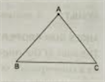Solution:

We get a triangle by joining the three non – collinear points A, B and C.

(i) The side opposite to $\angle C$ is AB.

(ii) The angle opposite to the side BC is $\angle A$.

(ii) The vertex opposite to the side CA is B.

(iv) The side opposite to the vertex B is AC.

QUESTION – 2: The measures of two angles of a triangle are $72^{\circ}\;and\;58^{\circ}$.

Find the measure of the third angle.

Solution:

The measure of two angles of a triangle are $72^{\circ}\;and\;58^{\circ}$.

Let the third angle be x.

Now, the sum of the measures of all the angles of a triangle is $180^{\circ}$.

Therefore, $x+72^{\circ}+58^{\circ}=180^{\circ}$

$\rightarrow x+130^{\circ}=180^{\circ}$

$\rightarrow x=180^{\circ}-130^{\circ}$

$\rightarrow x=50^{\circ}$

The measure of third angle of the triangle is $50^{\circ}$.

QUESTION – 3: The angles of a triangle are in the ratio 1: 3: 5. Find the measure of the third angle.

Solution:

The angles of a triangle are in the ratio 1:3:5.

Let the measures of the angles of the triangle be (1x), (3x) and (5x)

Sum of the measures of the angles of the triangle = $180^{\circ}$

Therefore, $1x+3x+5x=180^{\circ}$

=> 9x = $180^{\circ}$

=> x = $20^{\circ}$

1x = $20^{\circ}$

3x = $60^{\circ}$

5x = $100^{\circ}$

The measures of the angles are $20^{\circ},60^{\circ}\;and\;100^{\circ}$.

QUESTION – 4: One of the acute angles of a right triangle is $50^{\circ}$. Find the other acute angle.

Solution:

In a right angle triangle, one of the angles is $90^{\circ}$.

It is given that one of the acute angled of the right angled triangle is $50^{\circ}$.

We know that the sum of the measures of all the angles of a triangle is $180^{\circ}$.

Now, let the third angle be x.

Therefore, we have:

$90^{\circ}+50^{\circ}+x=180^{\circ}$

$\rightarrow 140^{\circ}+x=180^{\circ}$

$\rightarrow x=180^{\circ}-140^{\circ}$

$\rightarrow x=40^{\circ}$

The third acute angle is $40^{\circ}$.

QUESTION – 5: One of the angles of a triangle is $110^{\circ}$ and the other two angles are equal. What is the measure of each of these equal angles?

Solution: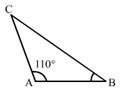Given:

$\angle A=110^{\circ} and \angle B=\angle C$

Now, the sum of the measures of all the angles of a triangle is $180^{\circ}$.

$\angle A+\angle B+\angle C=180^{\circ}$

=>$110^{\circ}+\angle B+\angle B=180^{\circ}$

$\rightarrow 110^{\circ}+2\angle B=180^{\circ}$

$\rightarrow 2\angle B=180^{\circ}-110^{\circ}$

$\rightarrow 2\angle B=70^{\circ}$

$\rightarrow \angle B=\frac{70^{\circ}}{2}$

$\rightarrow \angle B=35^{\circ}$

Therefore, $\angle C=35^{\circ}$

THE MEASURES OF THE THREE ANGLES:

$\angle A=110^{\circ},\angle B=35^{\circ},\angle C=35^{\circ}$

QUESTION – 6: If one angle of a triangle is equal to the sum of other two, show that the triangle is a right triangle.

Hint.$\angle A=\angle B+\angle C\rightarrow \angle A+\angle A=\angle A+\angle B+\angle C=180^{\circ}$

Solution:

Given:

$\angle A=\angle B+\angle C$

We know:

$\angle A+\angle B+\angle C=180^{\circ}$

$\rightarrow \angle B+\angle C+\angle B+\angle B=180^{\circ}$

$\rightarrow 2\angle B+2\angle C=180^{\circ}$

$\rightarrow 2\left ( \angle B+\angle C \right )=180^{\circ}$

$\rightarrow \angle B+\angle C =\frac{180^{\circ}}{2}$

$\rightarrow \angle B+\angle C =90^{\circ}$

Therefore, $\angle A=90^{\circ}$

This shows that the triangle is a right angled triangle.

QUESTION – 7: In a $\Delta ABC,\;if \;3\angle A=4\angle B=6\angle c$, calculate the angles.

Solution:

Let $3\angle A=4\angle B=6\;\angle C=x$

Then, we have:

$\angle A=\frac{x}{3},\angle B=\frac{x}{4},\angle C=\frac{x}{6}$

But, $\angle A+\angle B+\angle C=180^{\circ}$

Therefore, $\frac{x}{3}+\frac{x}{4}+\frac{x}{6}=180^{\circ}$

$or\;\frac{4x+3x+2x}{12}=180^{\circ}$

$or\;9x=180^{\circ}\times 12=2160^{\circ}$

$or\;x=240^{\circ}$

Therefore, $\angle A=\frac{240}{3}=80^{\circ},\angle B=\frac{240}{4}=60^{\circ},\angle C=\frac{240}{6}=40^{\circ}$

QUESTION – 8: Look at the figures given below. State for each triangle whether it is acute, right or obtuse.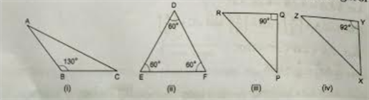Solution:

(i) It is an obtuse angle triangle as one angle is $130^{\circ}$, which is greater than $90^{\circ}$.

(ii) It is an acute angle triangle as all the angles in it are less than $90^{\circ}$.

(iii) It is a right angle triangle as one angle is $90^{\circ}$.

(iv) It is an obtuse angle triangle as one angle is $92^{\circ}$, which is greater than $90^{\circ}$.

QUESTION – 9: In the given figure some triangles have been given. State for each triangle whether it is scalene, isosceles or equilateral.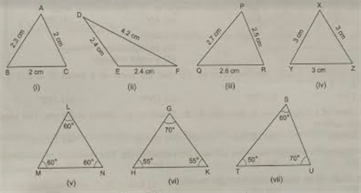Solution:

Equilateral Triangle: A triangle whose all three sides are equal in length and each of the three angles measures $60^{\circ}$.

Isosceles Triangle: A triangle whose two sides are equal in length and the angles opposite to them are equal to each other.

Scalene Triangle: A triangle whose all three sides and angles are unequal in the measure.

(i) Isosceles

AC = CB = 2 cm

(ii) Isosceles

DE = EF = 2.4 cm

(iii) Scalene

All sides are unequal.

(iv) Equilateral

XY = YZ = ZX = 3 cm

(v) Equilateral

All three angles are $60^{\circ}$.

(vi) Isosceles

Two angles are equal in measure.

(vii) Scalene

All the angles are unequal.

QUESTION – 10: Draw $\Delta ABC$. Take a point D on BC. Join AD.

How many triangles do you get?

Name them.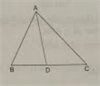Solution:

In $\Delta ABC$, if we take a point D on BC, then we get three triangles, namely $\Delta ADB,\Delta ADC\;and\;\Delta ABC$.

QUESTION – 11: Can a triangle have

(i) two right angles?

(ii) two obtuse angles?

(iii) two acute angles?

(iv) each angle more than $60^{\circ}$ ?

(v) each angle less than $60^{\circ}$ ?

(vi) each angle equal to $60^{\circ}$ ?

Solution:

(i) No

If the two angles are $90^{\circ}$ each, then the sum of two angles of a triangle will be $180^{\circ}$, which is not possible.

(ii) No

For example, let the two angles be $120^{\circ}$ and $150^{\circ}$. Then, their sum will be $270^{\circ}$, which cannot form a triangle.

(iii) Yes

For example, let the two angles be $50^{\circ}$ and $60^{\circ}$, which on adding, gives $110^{\circ}$. They can easily form a triangle whose third angle is $180^{\circ}-110^{\circ}=70^{\circ}$.

(iv) No

For example, let the two angles be $70^{\circ}$ and $80^{\circ}$, which on adding, gives $150^{\circ}$. They cannot form a triangle whose third angle is $180^{\circ}-110^{\circ}=70^{\circ}$, which is less than $60^{\circ}$.

(v) No

For example, let the two angles be $50^{\circ}$ and $40^{\circ}$, which on adding, gives $90^{\circ}$. Thus, they cannot form a triangle whose third angle is $180^{\circ}-90^{\circ}=90^{\circ}$, which is greater than $60^{\circ}$.

(vi) Yes

Sum of all angles = $60^{\circ}+60^{\circ}+60^{\circ}=180^{\circ}$.

QUESTION – 12: Fill in the blanks:

(i) A triangle has …….. sides, …….. angles and ……… vertices.

(ii) The sum of the angles of a triangle is ………. .

(iii) The sides of a scalene triangle are of ………… lengths.

(iv) Each angle of an equilateral triangle measures ………. .

(v) The angles opposite to equal sides of a triangle is called its ………… .

(vi) The sum of the lengths of the sides of a triangle is called its ……….. .

Solution:

(i) A triangle has $\underline{3}$ sides $\underline{3}$ angles and $\underline{3}$ vertices.

(ii) The sum of the angles of a triangle is $\underline{180}^{\circ}$.

(iii) The sides of a scalene triangle are of $\underline{different}$ lengths.

(iv) Each angle of an equilateral triangle measures $\underline{60}^{\circ}$.

(v) The angles opposite to equal sides of an isosceles triangle are $\underline{equal}$.

(vi) The sum of the lengths of the sides of a triangle is called its $\underline{perimeter}$.

Exercise – 16 B

OBJECTIVE QUESTIONS

Mark () against the correct answer in each of the following:

QUESTION – 1: How many parts does a triangle have?

(a) 2 (b) 3 (c) 6 (d) 9

Solution:

Correct option: (c)

A triangle has 6 parts: three sides and three angles.

QUESTION – 2: With the angles given below, in which case the construction of triangle is possible?

(a) $30^{\circ},60^{\circ},70^{\circ}$

(b) $50^{\circ},70^{\circ},60^{\circ}$

(c) $40^{\circ},80^{\circ},65^{\circ}$

(d) $72^{\circ},28^{\circ},90^{\circ}$

Solution:

Correct option: (b)

(a) Sum = $30^{\circ}+60^{\circ}+70^{\circ}=160^{\circ}$

This is not equal to the sum of all the angles of a triangle.

(b) Sum = $50^{\circ}+70^{\circ}+60^{\circ}=180^{\circ}$

Hence, it is possible to construct a triangle with these angles.

(c) Sum = $40^{\circ}+80^{\circ}+65^{\circ}=185^{\circ}$

This is not equal to the sum of all the angles of a triangle.

(d) Sum = $72^{\circ}+28^{\circ}+90^{\circ}=190^{\circ}$

This is not equal to the sum of all the angles of a triangle.

QUESTION – 3: The angles of a triangle are in the ratio 2: 3: 4. The largest angle is

(a) $60^{\circ}$ (b) $80^{\circ}$

(c) $76^{\circ}$ (d) $84^{\circ}$

Solution:

(b) $80^{\circ}$

Let the measures of the given angles be $\left ( 2x \right )^{\circ},\left ( 3x \right )^{\circ}\;and\;\left ( 4x \right )^{\circ}$.

Therefore, $\left ( 2x \right )^{\circ}+\left ( 3x \right )^{\circ}+\left ( 4x \right )^{\circ}=180^{\circ}$

$\rightarrow \left ( 9x \right )^{\circ}=180^{\circ}$

=> x = 180/9

=> x = $20^{\circ}$

Therefore, $2x=40^{\circ},3x=60^{\circ},4x=80^{\circ}$

Hence, the measures of the angles of the triangle are $40^{\circ},60^{\circ},80^{\circ}$.

Thus, the largest angle is $80^{\circ}$.

QUESTION – 4: The two angles of a triangle are complementary. The third angle is

(a) $60^{\circ}$ (b) $45^{\circ}$

(c) $36^{\circ}$ (d) $90^{\circ}$

Solution:

Correct option: (d)

The measure of two angles are complimentary if their sum is $90^{\circ}$.

Let the two angles be x and y, such that x + y = $90^{\circ}$.

Let the third angle be z.

Now, we know that the sum of all the angles of a triangle is $180^{\circ}$.

x + y + z = $180^{\circ}$.

=> $90^{\circ}$ + z = $180^{\circ}$

=> z = $180^{\circ}-90^{\circ}$

= $90^{\circ}$

The third angle is $90^{\circ}$.

QUESTION – 5: One of the base angles of an isosceles triangle is $70^{\circ}$. The vertical angle is

(a) $60^{\circ}$ (b) $80^{\circ}$

(c) $40^{\circ}$ (d) $35^{\circ}$

Solution:

Correct option: (c)

Let $\angle A=70^{\circ}$

The triangle is an isosceles triangle.

We know that the angles opposite to the equal sides of an isosceles triangle are equal.

Therefore, $\angle B=70^{\circ}$

We need to find the vertical angle $\angle C$.

Now, sum of all the angles of a triangle is $180^{\circ}$.

$\angle A+\angle B+\angle C=180^{\circ}$

$\rightarrow 70^{\circ}+70^{\circ}+\angle C = 180^{\circ}$

$\rightarrow 140^{\circ}+\angle C = 180^{\circ}$

$\rightarrow \angle C = 180^{\circ}-140^{\circ}$

$\rightarrow \angle C = 40^{\circ}$

QUESTION – 6: A triangle having sides of different lengths is called

(a) an isosceles triangle (b) an equilateral triangle

(c) a scalene triangle (d) a right triangle

Solution:

Correct option: (c)

A triangle having sides of different lengths is called a scalene triangle.

QUESTION – 7: In an isosceles $\Delta ABC$, the bisectors of $\angle B\;and\;\angle C$ meet at point O. If $\angle A=40^{\circ} \;then \;\angle BOC=?$

(a) $110^{\circ}$ (b) $70^{\circ}$

(c) $130^{\circ}$ (d) $150^{\circ}$

Solution:

Correct option: (a)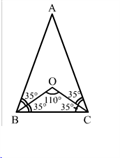In the isosceles ABC, the bisectors of $\angle B\;and\;\angle C$meet at point O.

Since the triangle is isosceles, the angles opposite to the equal sides are equal.

Practise This Question

The bones of the birds are ___________ and ___________.